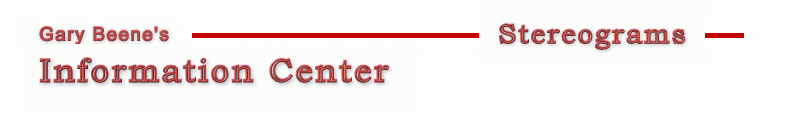Stereo Algorithms - Hidden Surface Removal This page shows how to modify the basic stereogram code to incorporate a hidden surface removal algorithm. Basic Stereogram with Hidden Surface Removal Source Code: ```1 Dim x As Long, y As Long, maxX As Long, maxY As Long, same() As Long 'basic 2 Dim z() As Single, mu As Single, E As Long, DPI As Long, s As Long 'basic 3 Dim right As Long, left As Long, w As Single 'basic 4 Dim L As Long 'smart links 5 Dim zt As Single, t As Long, visible As Long 'hidden surface removal 6 DPI = 72: E = 2.5 * DPI: mu = 0.2: w = 0.5 w = whitespace: mu = depth 7 maxX = picDepth.ScaleWidth: maxY = picDepth.ScaleHeight 8 ReDim same(maxX), z(maxX, maxY): Randomize 9 For y = 0 To maxY 10 For x = 0 To maxX - 1 '1 scanline 11 z(x, y) = picDepth.Point(x, y) / 16777215 'initialize 12 same(x) = x 'initialize 13 s = (1 - mu * z(x, y)) * E / (2 - mu * z(x, y)) 'image plane separation 14 left = x - (s / 2) 'left link position 15 right = left + s 'right link position 16 If 0 <= left And right < maxX Then 17 t = 1 18 Do 19 zt = z(x, y) + 2 * (2 - mu * z(x, y)) * t / (mu * E) 20 visible = (z(x - t, y) < zt) And (z(x + t, y) < zt) 21 t = t + 1 22 Loop While visible And (zt < 1) 23 If visible Then same(left) = right 'create link 24 End If 25 Next x 26 For x = maxX - 1 To 0 Step -1 'set colors 27 If same(x) = x Then 28 picOut.PSet (x, y), Int(Rnd + w) * vbWhite 'random Rnd*vbwhite for color 29 Else 30 picOut.PSet (x, y), picOut.Point(same(x), y) 'use link color 31 End If 32 Next x 33 Next y ``` Code Discussion The hidden surface removal code, found in lines 17-23, works in the following way ...